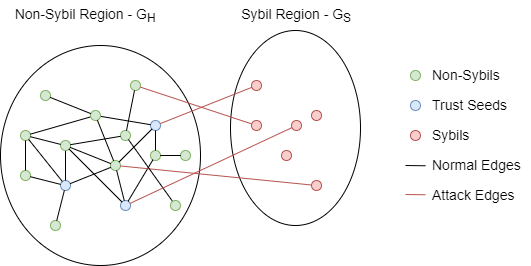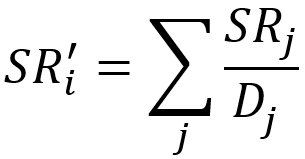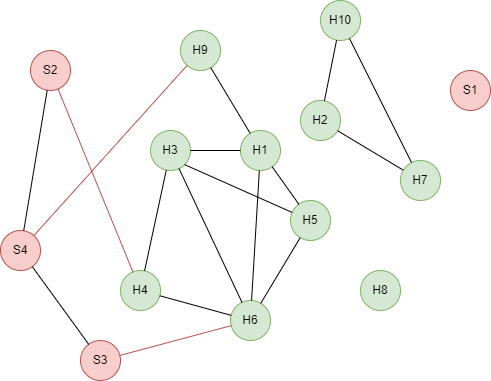# Change Nickname

Current Nickname:

Certifications

Certificate Issued at Valid until Serial No. File
Serial No. Valid until

Not having one? Apply now! >>>

Invoice

ProductName CreateTime ID Price File
ProductName CreateTime ID Price File
v4.0
Search
中文EN
v4.0

# SybilRank

## Overview

SybilRank is an algorithm that ranks the trustworthiness of nodes in a multi-community structure network by short random walk through power iteration. The word Sybil originally refers to the fake account on the Online Social Network (OSN). The surge in popularity of OSNs has accompanied by the increasing Sybil attacks and abuses, such as sending spam messages to other accounts, maliciously increasing the number of clicks on advertisements or web links, and crawling private account information to act as water army or commit cyber violence.

SybilRank algorithm was proposed by Qiang Cao et al. in 2012. The algorithm has good calculation cost-performance ratio and large graph scalability (with parallelizability), which could help social network platforms or related enterprises to locate fake accounts more efficiently.

Related material of the algorithm:

## Basic Concept

### Trust Seeds

Trust seeds in the SybilRank algorithm refer to the user-specified trustworthy non-Sybil nodes (accounts owned by real humans).

### Threat Model

Each node that participates in the SybilRank algorithm corresponds to one account in the OSN, and each edge (undirected) corresponds to a bilateral social relationship between two accounts. SybilRank algorithm divides all accounts into two disjoint sets `H` and `S`, representing non-Sybil and Sybil users respectively; and it denotes edges between `H` and `S` as attacks launched from Sybils to non-Sybils, the number of attack edges should be much less than the number of edges between non-Sybils. This is the Threat Model of SybilRank.

Please note, the subgraph `G(H)` formed by all non-Sybils `H` and edges between them cannot be bipartite. In this case, the landing probability of random walks at each trust seed is proportional to the node's degree.

The diagram below is an example of threat model:### Landing Probability / Node's Trust

Early-terminated power iteration is used by the random walk of SybilRank. Due to the limited attack edges between `H` and `S`, the random walk departs from trust seeds would land at non-Sybils with a greater probability before the whole graph stabilizes, and we call this probability as Landing Probability, or you may consider it as the node's trust. Ranking the trust of each node, the less trust the more likely the node is Sybil.

A total trust should be given when initializing the algorithm, which is first evenly distributed among all trust seeds. During each iteration, each node's trust is evenly distributed among all neighbors of the node along the edges of the node (edge direction ignored) while the total trust of the whole graph always remains the same. Thus, the trust of node `i` after each iteration is:where `j` is any neighbor of node `i`, the number of `i`'s neighbors equals to `i`'s degree, i.e. each self-loop edge of node is counted as 2 neighbors; `D(j)` is degree of `j`.

In the original paper, the algorithm normalizes the node's trust before the final ranking (Degree-Normalization), that is, divided the trust by the node degree. Ultipa's SybilRank algorithm simplifies this by using the trust obtained after iterations directly as the basis for ranking.

When the algorithm begins, initial trusts for all trust seeds are assigned based on the algorithm parameters; during each iteration, trust of each node is calculated and updated. Iterating and looping by this rule until the number of iterations reaches the limit.

### Mixing Time

Although the same power iteration is used to pass trust/score, PageRank algorithm aims to achieve a stable score distribution for the whole directed graph, while SybilRank aims to get a steady trust distribution for only the undirected `G(H)`. The number of walking steps required for the latter, also known as Mixing Time, is the number of iterations needed, which usually is the logarithm `log(number of nodes)` based on 2 (rounded up). In practice, the mixing time of `G(H)` is affected by many factors, so `log(number of nodes)` is only a reference, but it must be less than the mixing time of the whole graph, which is the characteristic of this iteration - early termination.

## Special Case

### Lonely Node, Disconnected Graph

Since no node is connected with lonely node by edge, the trust of lonely node is 0 if it is not specified as trust seed; when lonely node is specified as one of the trust seeds, the trust of lonely node equals to total trust divided by the number of trust seeds.

There is no trust transfer between different connected components in disconnected graph.

### Self-loop Edge

Self-loop edge is regarded as one outbound edge and one inbound edge, i.e. each self-loop edge is counted as two edges for the node.

### Directed Edge

For directed edges, SybilRank algorithm ignores the direction of edges but calculates them as undirected edges.

## Results and Statistics

Take the social network graph below as an example, run the SybilRank algorithm, specify [H1,H2,H3] as trust seeds, total trust as 100, `log14` = `3.8074``4` iterations are executed:Algorithm results: Calculate trust for each node, return `_id`, `rank` or `_uuid`, `rank` according to the execution method

_uuid _id rank
11 S1 0.0000000
8 H8 0.0000000
9 H9 3.7355320
12 S2 3.8078699
13 S3 4.0046301
14 S4 6.1284719
4 H4 6.8836799
5 H5 7.6562500
7 H7 10.416666
10 H10 10.416666
3 H3 10.691550
1 H1 11.114004
2 H2 12.500000
6 H6 12.644675

Algorithm statistics: N/A

## Command and Configuration

• Command: `algo(sybil_rank)`
• Configurations for the parameter `params()`:
Name
Type
Default
Specification
Description
total_trust float / > 0 Total trust of the graph, which would be evenly distributed among all trust seeds; all nodes to be specified if not set
trust_seeds []`_uuid` / / UUID of trust seeds, it is suggested to assign trust seeds for every community
loop_num int 5 > 0 Number of iterations, the reference is the logarithm `log(number of nodes)` based on 2 (rounded up)
limit int -1 >=-1 Number of results to return; return all results if sets to -1 or not set

Example: Run the SybilRank algorithm, specify UUID = 1,2,3 as trust seeds, total trust as 100, iterate 4 rounds

``````algo(sybil_rank).params({
total_trust: 100,
trust_seeds: [1,2,3],
loop_num: 4
}) as rank return rank
``````

## Algorithm Execution

#### 1. File Writeback

Configuration Data in Each Row
filename `_id`,`rank`

Example: Run the SybilRank algorithm, specify UUID = 1,2,3 as trust seeds, total trust as 100, iterate 4 rounds, write the algorithm results back to file named sybilRank

``````algo(sybil_rank).params({
total_trust: 100,
trust_seeds: [1,2,3],
loop_num: 4
}).write({
file:{
filename: "sybilRank"
}
})
``````

#### 2. Property Writeback

Configuration Writeback Content Type Data Type
property `rank` Node property `float`

Example: Run the SybilRank algorithm, specify UUID = 1,2,3 as trust seeds, total trust as 100, iterate 4 rounds, write the algorithm results back to node property named trust

`````` algo(sybil_rank).params({
total_trust: 100,
trust_seeds: [1,2,3],
loop_num: 4
}).write({
db:{
property: "trust"
}
})
``````

#### 3. Statistics Writeback

This algorithm has no statistics.

### Direct Return

Alias Ordinal Type Description Column Name
0 []perNode Node and its trust `_uuid`, `rank`

Example: Run the SybilRank algorithm, specify UUID = 1,2,3 as trust seeds, total trust as 100, iterate 4 rounds, define algorithm results as alias named rank, and return the result

``````algo(sybil_rank).params({
total_trust: 100,
trust_seeds: [1,2,3],
loop_num: 4
}) as rank return rank
``````

### Streaming Return

Alias Ordinal Type Description Column Name
0 []perNode Node and its trust `_uuid`, `rank`

Example: Run the SybilRank algorithm, specify UUID = 1,2,3 as trust seeds, total trust as 100, iterate 4 rounds, return the most suspicious 4 nodes with their property name and score

``````algo(sybil_rank).params({
total_trust: 100,
trust_seeds: [1,2,3],
loop_num: 4,
order: "asc",
limit: 10
}).stream() as results
find().nodes({_uuid == results._uuid}) as nodes
return table(nodes.name, results.rank)
``````

### Real-time Statistics

This algorithm has no statistics.

## Algorithm Efficiency

Computation complexity of the SybilRank algorithm is `O(n log n)`, and it is not related with the number of trust seeds